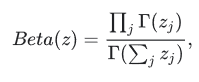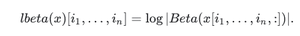Open in App
Not now

# Python – tensorflow.math.lbeta()

• Last Updated : 14 Sep, 2021

TensorFlow is open-source Python library designed by Google to develop Machine Learning models and deep learning  neural networks.

lbeta() is used to compute ln(|Beta(x)|). It reduces the tensor along the last dimension. If one-dimensional z is [z1, …, zk], then Beta(z) is defined asIf x is n+1 dimensional tensor with shape [N1 , . . ., Nn , k], last dimension is treated as z vector and,If z = [u, v] then traditional bivariate beta function is defined asSyntax: tensorflow.math.lbeta( x, name)

Parameters:

• x: It’s the input tensor with rank n+1 where n>=0. Allowed dtypes  are float, or double.
• name(optional): It defines the name for the operation.

Returns:

It returns the logarithm of |Beta(x)| reducing along the last dimension.

Example 1:

## Python3

 `# Importing the library` `import` `tensorflow as tf`   `# Initializing the input tensor` `a ``=` `tf.constant([[``7``, ``8``], [``13``, ``11``]], dtype ``=` `tf.float64)`   `# Printing the input tensor` `print``(``'a: '``, a)`   `# Calculating the result` `res ``=` `tf.math.lbeta(x ``=` `a)`   `# Printing the result` `print``(``'Result: '``, res)`

Output:

```a:  tf.Tensor(
[[ 7.  8.]
[13. 11.]], shape=(2, 2), dtype=float64)
Result:  tf.Tensor([-10.08680861 -16.5150485 ], shape=(2, ), dtype=float64)```

Example 2:

## Python3

 `# Importing the library` `import` `tensorflow as tf`   `# Initializing the input tensor` `a ``=` `tf.constant([``7``, ``8``, ``13``, ``11``], dtype ``=` `tf.float64)`   `# Printing the input tensor` `print``(``'a: '``, a)`   `# Calculating the result` `res ``=` `tf.math.lbeta(x ``=` `a)`   `# Printing the result` `print``(``'Result: '``, res)`

Output:

```a:  tf.Tensor([ 7.  8. 13. 11.], shape=(4, ), dtype=float64)
Result:  tf.Tensor(-52.77215897270088, shape=(), dtype=float64)```

My Personal Notes arrow_drop_up
Related Articles